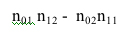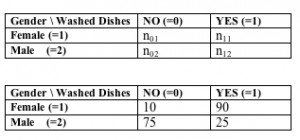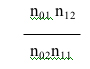# Baby Steps to Logistic regressionGoing from the phi coefficient to odds-ratios. Remember the numerator for the phi coefficient waswell, the odds ratio is the same two numbers DIVIDED rather than subtracted. You might think it is four numbers, but really it is not. The first number is the product of the diagonal cells (see below). The second number is the product of the off-diagonal cells. Let’s take a look at our data again, first in symbolic form and then the actual numbers.So the odds of a woman doing the dishes are 9:1 , that is for every one woman who doesn’t do the dishes, there are nine who do. The odds of a man doing the dishes are 1:3, that is, for every three men who don’t do the dishes, there is one who does.

Here is our formula for the odds ratio:=   (10*25)  /(75*90)  =   1/27

The odds of a man doing the dishes (1/3) are one-twenty-seventh the odds of a woman doing them (9/1).

Tomorrow, I will try to find the time  to explain how this is intimately related to logistic regression.

But for now, I am going to go home, and, no doubt, eventually do the dishes.